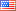# Revision history [back]

### 3D graphics bug?

Hi,

When I use the following code and I change the values of phi or theta, the previous red dot(point of projection) remains on the image and the new dot is added onto the image, giving two red dots. If I repeatedly change the values then more red dots appear. This problem does not occur if I get rid of the polygon inside the sphere. I'm not sure why this is happening...

S = sphere(size=10,color="yellow",opacity=0.5) north_pole = point3d((0,0,10), color="blue") r = 10 @interact def _(theta = slider(0,2pi,pi/20, default = pi/3, label="theta"), phi = slider(0,2pi,pi/20, default = pi/4, label="phi")): point_of_projection = point3d((rsin(theta)cos(phi),rsin(theta)sin(phi),r*cos(theta)), color="red")

## show(S + north_pole + point_of_projection)2 No.2 RevisionShashank1887 ●28 ●47 ●82

### 3D graphics bug?

Hi,

When I use the following code and I change the values of phi or theta, the previous red dot(point of projection) remains on the image and the new dot is added onto the image, giving two red dots. If I repeatedly change the values then more red dots appear. This problem does not occur if I get rid of the polygon inside the sphere. I'm not sure why this is happening...

S = sphere(size=10,color="yellow",opacity=0.5)
north_pole = point3d((0,0,10), color="blue")
r = 10
@interact
def _(theta = slider(0,2pi,pi/20, slider(0,2*pi,pi/20, default = pi/3, label="theta"),
phi = slider(0,2pi,pi/20, slider(0,2*pi,pi/20, default = pi/4, label="phi")):
point_of_projection = point3d((rsin(theta)cos(phi),rsin(theta)sin(phi),r*cos(theta)), color="red")point3d((r*sin(theta)*cos(phi),r*sin(theta)*sin(phi),r*cos(theta)), color="red")
show(S + north_pole + point_of_projection)point_of_projection)3 No.3 Revision

### 3D graphics bug?

Hi,

When I use the following code and I change the values of phi or theta, the previous red dot(point of projection) remains on the image and the new dot is added onto the image, giving two red dots. If I repeatedly change the values then more red dots appear. This problem does not occur if I get rid of the polygon inside the sphere. I'm not sure why this is happening...

Works:

S = sphere(size=10,color="yellow",opacity=0.5)
north_pole = point3d((0,0,10), color="blue")
r = 10
@interact
def _(theta = slider(0,2*pi,pi/20, default = pi/3, label="theta"),
phi = slider(0,2*pi,pi/20, default = pi/4, label="phi")):
point_of_projection = point3d((r*sin(theta)*cos(phi),r*sin(theta)*sin(phi),r*cos(theta)), color="red")
show(S + north_pole + point_of_projection)


Doesn't work:

Z = Polyhedron([[2,3,3],[3,0,3],[2,6,1],[1,0,1],[6,7,2]])
Z_set_as_graphics = Z.show()
S = sphere(size=10,color="yellow",opacity=0.5)
north_pole = point3d((0,0,10), color="blue")
r = 10
@interact def _(theta = slider(0,2pi,pi/20, default = pi/3, label="theta"), phi = slider(0,2pi,pi/20, default = pi/4, label="phi")):
point_of_projection = point3d((rsin(theta)cos(phi),rsin(theta)sin(phi),r*cos(theta)), color="red")
show(Z_set_as_graphics + S + north_pole + point_of_projection)4 No.4 Revision

### 3D graphics bug?

Hi,

When I use the following code and I change the values of phi or theta, the previous red dot(point of projection) remains on the image and the new dot is added onto the image, giving two red dots. If I repeatedly change the values then more red dots appear. This problem does not occur if I get rid of the polygon inside the sphere. I'm not sure why this is happening...

Works:

S = sphere(size=10,color="yellow",opacity=0.5)
north_pole = point3d((0,0,10), color="blue")
r = 10
@interact
def _(theta = slider(0,2*pi,pi/20, default = pi/3, label="theta"),
phi = slider(0,2*pi,pi/20, default = pi/4, label="phi")):
point_of_projection = point3d((r*sin(theta)*cos(phi),r*sin(theta)*sin(phi),r*cos(theta)), color="red")
show(S + north_pole + point_of_projection)


Doesn't work:

Z = Polyhedron([[2,3,3],[3,0,3],[2,6,1],[1,0,1],[6,7,2]])
Z_set_as_graphics = Z.show()
S = sphere(size=10,color="yellow",opacity=0.5)
north_pole = point3d((0,0,10), color="blue")
r = 10
@interact  def _(theta = slider(0,2pi,pi/20, default = pi/3, label="theta"), phi = slider(0,2pi,pi/20, default = pi/4, label="phi")):
point_of_projection = point3d((rsin(theta)cos(phi),rsin(theta)sin(phi),r*cos(theta)), color="red")
show(Z_set_as_graphics + S + north_pole + point_of_projection)5 No.5 Revision

### 3D graphics bug?

Hi,

When I use the following code and I change the values of phi or theta, the previous red dot(point of projection) remains on the image and the new dot is added onto the image, giving two red dots. If I repeatedly change the values then more red dots appear. This problem does not occur if I get rid of the polygon inside the sphere. I'm not sure why this is happening...

Works:

S = sphere(size=10,color="yellow",opacity=0.5)
north_pole = point3d((0,0,10), color="blue")
r = 10
@interact
def _(theta = slider(0,2*pi,pi/20, default = pi/3, label="theta"),
phi = slider(0,2*pi,pi/20, default = pi/4, label="phi")):
point_of_projection = point3d((r*sin(theta)*cos(phi),r*sin(theta)*sin(phi),r*cos(theta)), color="red")
show(S + north_pole + point_of_projection)


Doesn't work:

Z = Polyhedron([[2,3,3],[3,0,3],[2,6,1],[1,0,1],[6,7,2]])
Z_set_as_graphics = Z.show()
S = sphere(size=10,color="yellow",opacity=0.5)
north_pole = point3d((0,0,10), color="blue")
r = 10
@interact
def _(theta = slider(0,2pi,pi/20, slider(0,2*pi,pi/20, default = pi/3, label="theta"), phi = slider(0,2pi,pi/20, slider(0,2*pi,pi/20, default = pi/4, label="phi")):
point_of_projection = point3d((rsin(theta)cos(phi),rsin(theta)sin(phi),r*cos(theta)), point3d((r*sin(theta)*cos(phi),r*sin(theta)*sin(phi),r*cos(theta)), color="red")
show(Z_set_as_graphics + S + north_pole + point_of_projection)